# 排序，搜索，算法模式，算法复杂度 | 数据结构与算法综合笔记### 排序算法

• 先创建一个数组来表示待排序和搜索的数据结构
``````function ArrayList(){
var array = []; //将项存储在数组中

this.insert = function(item){ //插入方法来向数据结构中添加元素
array.push(item);
};

this.toString = function(){ //来拼接数组中的所有元素至一个单一的字符串
return array.join();
};
}
// join方法拼接数组元素至一个字符串，并返回该字符串

### 冒泡排序

• 冒泡排序在运行时间的角度来看，是最差的。

``````this.bubbleSort = function(){
var length = array.length; // 用来存储数组的长度

for (var i=0; i<length; i++){
// 会从数组的第一位迭代至最后一位，它控制了在数组中经过多少轮排序
// 应该是数组中每项都经过一轮，轮数和数组长度一致
for (var j=0; j<length-1; j++ ){
//内循环将从第一位迭代至倒数第二位
//内循环实际上进行当前项和下一项的比较
if (array[j] > array[j+1]){
swap(array, j, j+1); //{5}
}
}
}
};

// 声明swap函数
// 一个私有函数
var swap = function(array, index1, index2){
var aux = array[index1];
array[index1] = array[index2];
array[index2] = aux;
};
// 我们用一个中间值来存储某一交换项的值

ES6写法：

``````[array[index1], array[index2]] = [array[index2], array[index1]];

``````this.modifiedBubbleSort = function(){
var length = array.length;

for (var i=0; i<length; i++){
for (var j=0; j<length-1-i; j++ ){ //避免内循环中所有不必要的比较
if (array[j] > array[j+1]){
swap(j, j+1);
}
}
}
};

### 选择排序(一种原址比较排序算法)

``````this.selectionSort = function(){
var length = array.length, indexMin;

for (var i=0; i<length-1; i++){
indexMin = i;
for (var j=i; j<length; j++){
if(array[indexMin]>array[j]){
indexMin = j;
}
}
if (i !== indexMin){
swap(i, indexMin);
}
}
};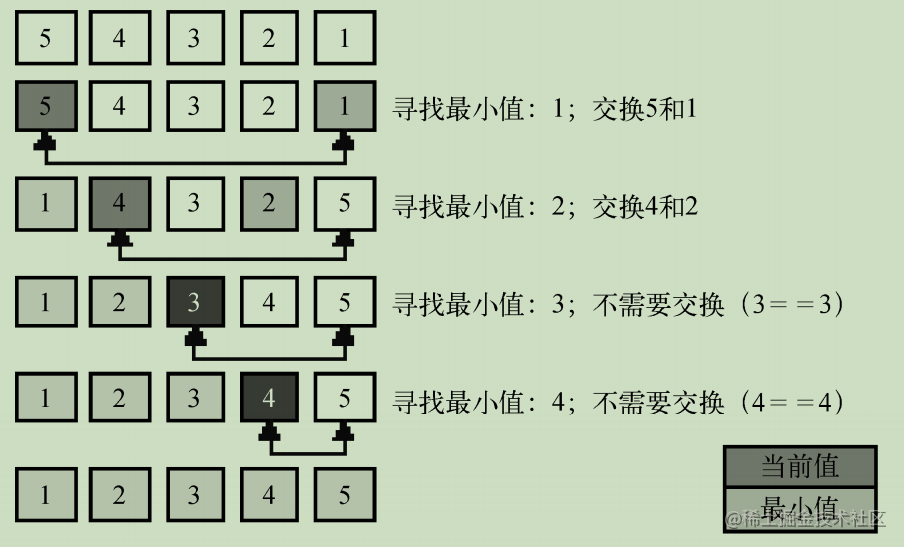### 插入排序

``````this.insertionSort = function(){
var length = array.length, j, temp;

for (var i=1; i<length; i++){
j = i;
temp = array[i];
while (j>0 && array[j-1] > temp){
array[j] = array[j-1];
j--;
}
array[j] = temp;
}
};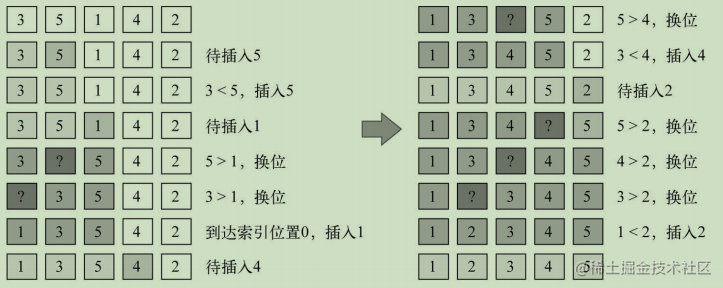### 归并排序

• 归并排序是一种分治算法
• 归并排序也是递归的
``````this.mergeSort = function(){
array = mergeSortRec(array);
};

``````// 归并排序将一个大数组转化为多个小数组直到只有一个项
var mergeSortRec = function(array){
var length = array.length;

if(length === 1) { //判断数组的长度是否为1
return array; //返回这个长度为1的数组
}

var mid = Math.floor(length / 2), //如果数组长度比1大，那么我们得将其分成小数组

left = array.slice(0, mid),
//left数组由索引0至中间索引的元素组成

right = array.slice(mid, length);
//right数组由中间索引至原始数组最后一个位置的元素组成

return merge(mergeSortRec(left), mergeSortRec(right)); //将数组分成两个小数组

};

``````// merge函数接受两个数组作为参数
// 并将它们归并至一个大数组
var merge = function(left, right){
var result = [], // 声明归并过程要创建的新数组
il = 0,
ir = 0;

while(il < left.length && ir < right.length) { // 迭代两个数组
// 比较来自left数组的项是否比来自right数组的项小
if(left[il] < right[ir]) {
result.push(left[il++]);
// 将该项从left数组添加至归并结果数组，并递增迭代数组的控制变量
} else{
result.push(right[ir++]);
// 从right数组添加项并递增相应的迭代数组的控制变量
}
}

while (il < left.length){ // {11}
result.push(left[il++]);
}

while (ir < right.length){ // {12}
result.push(right[ir++]);
}

return result; // {13}
};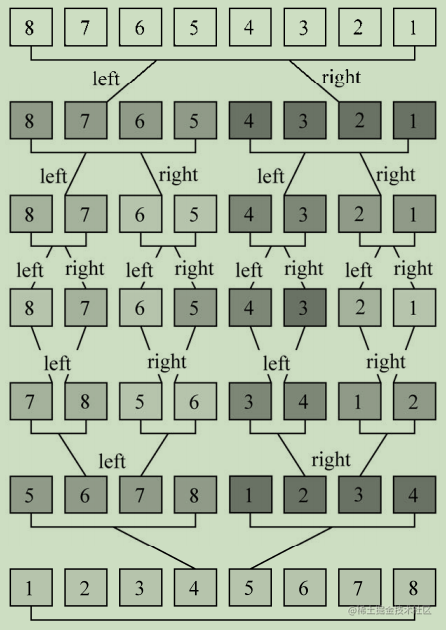### 快速排序

• 从数组中选择中间一项作为主元
• 创建两个指针，左边一个指向数组第一个项，右边一个指向数组最后一个项
• 移动左指针直到我们找到一个比主元大的元素
• 移动右指针直到找到一个比主元小的元素

``````this.quickSort = function(){
quick(array, 0, array.length - 1);
};

``````var quick = function(array, left, right){

var index; //该变量能帮助我们将子数组分离为较小值数组和较大值数组
if (array.length > 1) { //因为只有一个元素的数组必然是已排序了的
index = partition(array, left, right); //。partition函数返回值将赋值给index

if (left < index - 1) { //如果子数组存在较小值的元素
quick(array, left, index - 1); //对该数组重复这个过程
}
if (index < right) { //对存在较大值的子数组 如果存在子数组存在较大值
quick(array, index, right); //对该数组重复这个过程
}
}

};

• 划分过程

1.选择主元

``````var partition = function(array, left, right) {

var pivot = array[Math.floor((right + left) / 2)], //选择中间项作为主元
i = left, //初始化两个指针 初始化为数组第一个元素
j = right; //初始化两个指针 初始化为数组最后一个元素

while (i <= j) { //只要left和right指针没有相互交错就执行划分操作
while (array[i] < pivot) { //移动left指针直到找到一个元素比主元大
i++;
}
while (array[j] > pivot) { //移动right指针直到我们找到一个元素比主元小
j--;
}
if (i <= j) { //当左指针指向的元素比主元大且右指针指向的元素比主元小
// 左指针索引没有右指针索引大 左项比右项大
swap(array, i, j); //交换它们，然后移动两个指针
i++;
j--;
}
}
return i;
};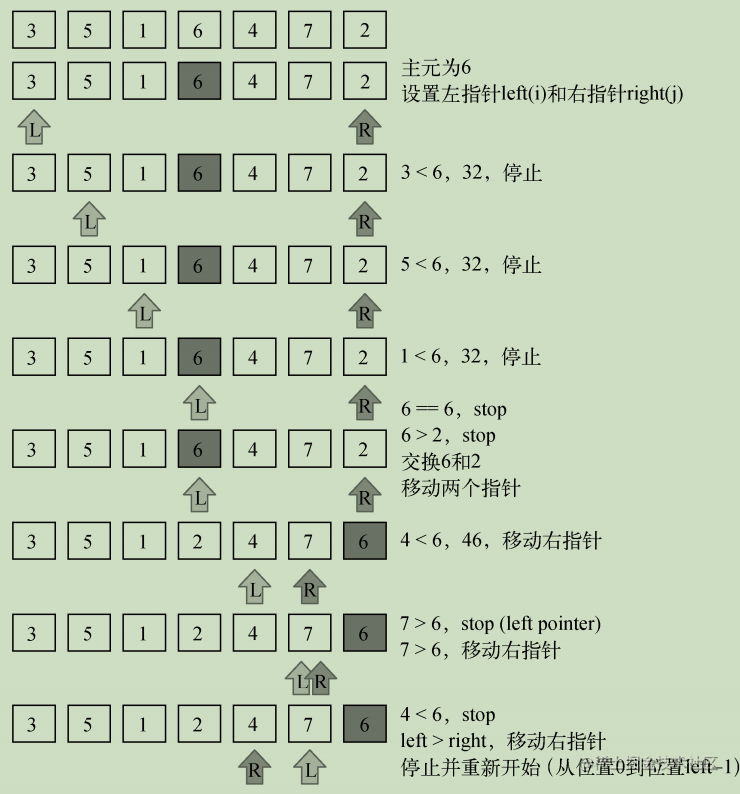• 下面的示意图展示了对有较小值的子数组执行的划分操作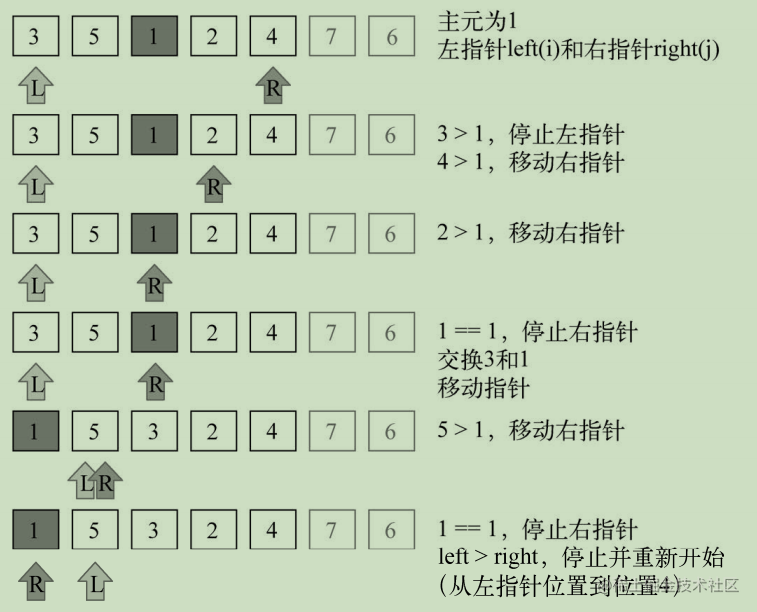• 继续创建子数组，请看下图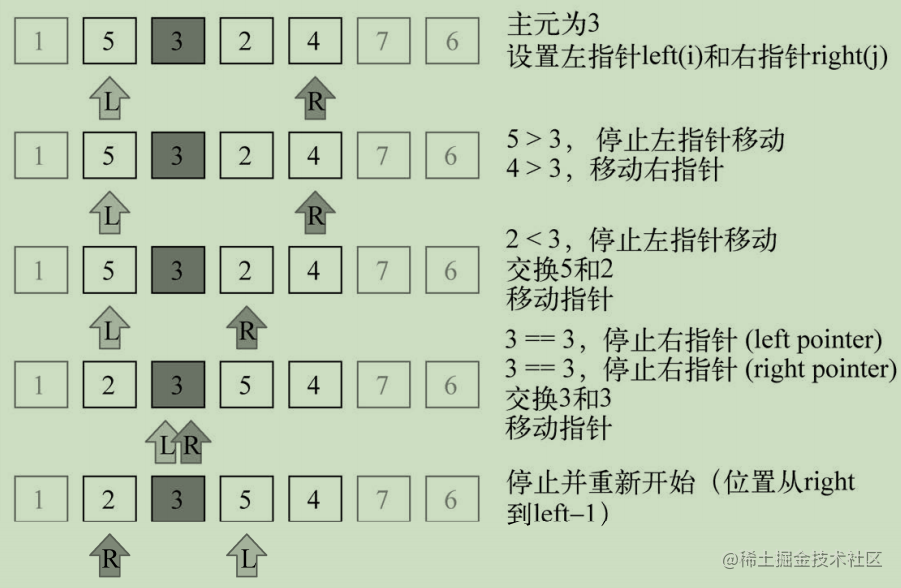• 继续进行划分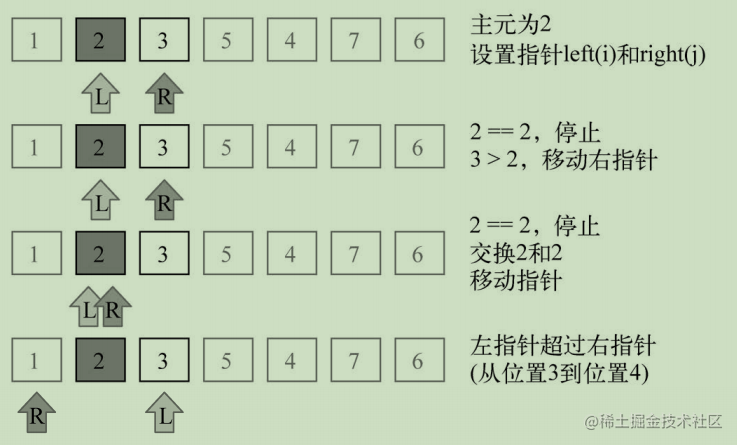• 继续进行划分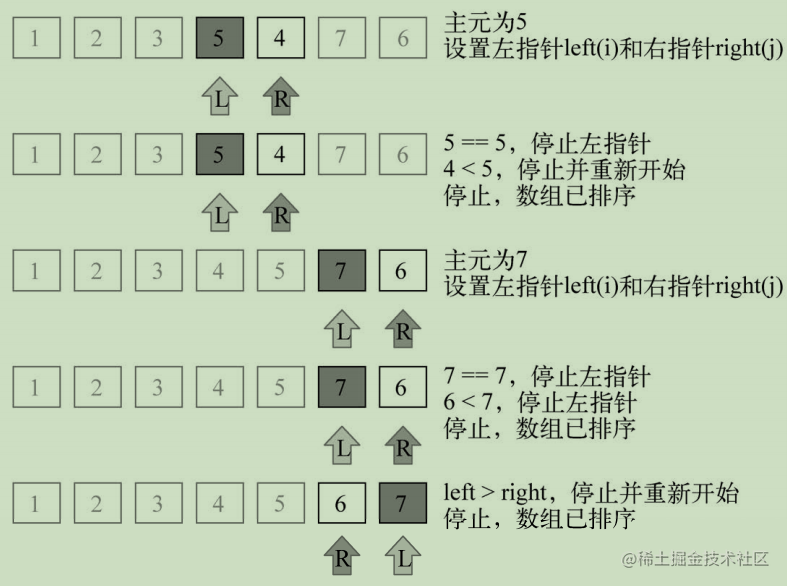### 堆排序

• 一种很高效的算法
• 把数组当作二叉树来排序而得名

1.索引0是树的根节点；

2.除根节点外，任意节点N的父节点是N/2；

3.节点L的左子节点是2*L；

4.节点R的右子节点是2*R+1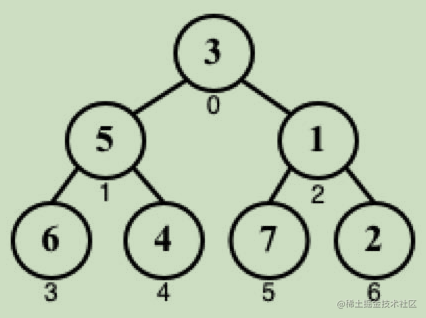``````this.heapSort = function() {

var heapSize = array.length;

buildHeap(array); //构造一个满足array[parent(i)] ≥ array[i]的堆结构

while (heapSize > 1) {
heapSize--;

swap(array, 0, heapSize); //交换堆里第一个元素和最后一个元素的位置

heapify(array, heapSize, 0);
//找到当前堆的根节点（较小的值），重新放到树的底部
}

};

`buildHeap`函数实现如下

``````var buildHeap = function(array){

var heapSize = array.length;

for (var i = Math.floor(array.length / 2); i >= 0; i--) {
heapify(array, heapSize, i);
}

};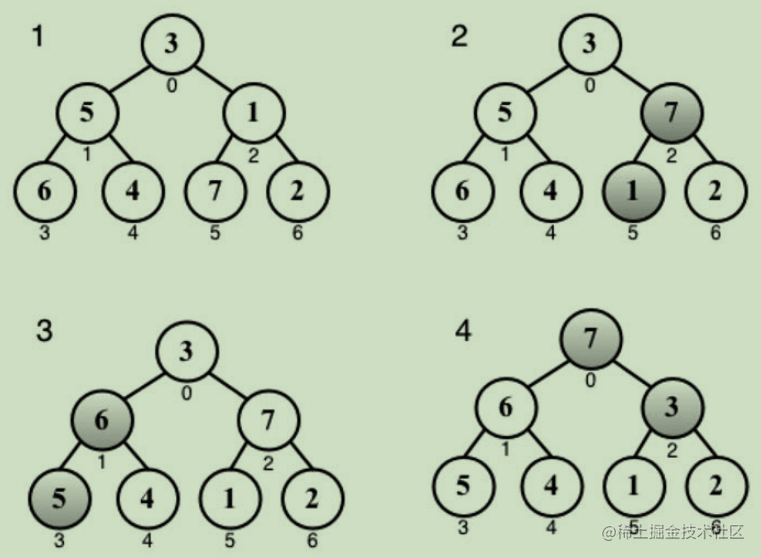``````var heapify = function(array, heapSize, i){

var left = i * 2 + 1,
right = i * 2 + 2,
largest = i;

if (left < heapSize && array[left] > array[largest]) {
largest = left;
}

if (right < heapSize && array[right] > array[largest]) {
largest = right;
}

if (largest !== i) {
swap(array, i, largest);
heapify(array, heapSize, largest);
}

};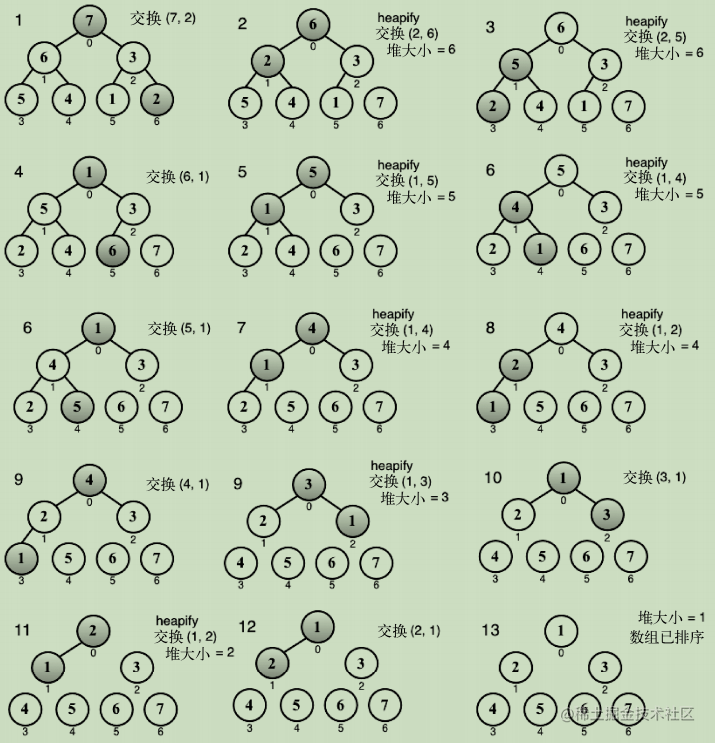1.计数排序

2.桶排序

3.基数排序

### 搜索算法-顺序搜索

• 顺序或线性搜索是最基本的搜索算法
• 将每一个数据结构中的元素和我们要找的元素做比较

``````this.sequentialSearch = function(item){
for (var i=0; i<array.length; i++){ //顺序搜索迭代整个数组
if (item === array[i]) //将每个数组元素和搜索项作比较
return i; //搜索成功
// 返回值可以是该搜索项本身，或是true，又或是搜索项的索引
}
}
return -1; //没有找到该项，则返回-1 表示该索引不存在
};

### 搜索算法-二分搜索

``````this.binarySearch = function(item){
this.quickSort(); //需要先将数组排序
var low = 0, //  在数组排序之后，我们设置low和high指针
high = array.length - 1,
mid, element;

while (low <= high){ //当low比high小时

mid = Math.floor((low + high) / 2);
element = array[mid];

if (element < item) {
//比较选中项的值和搜索值
low = mid + 1;
} else if (element > item) {
high = mid - 1;
} else {
return mid;
}
}

return -1; //我们计算得到中间项索引并取得中间项的值
//此处如果low比high大，则意思是该待搜索值不存在并返回-1
};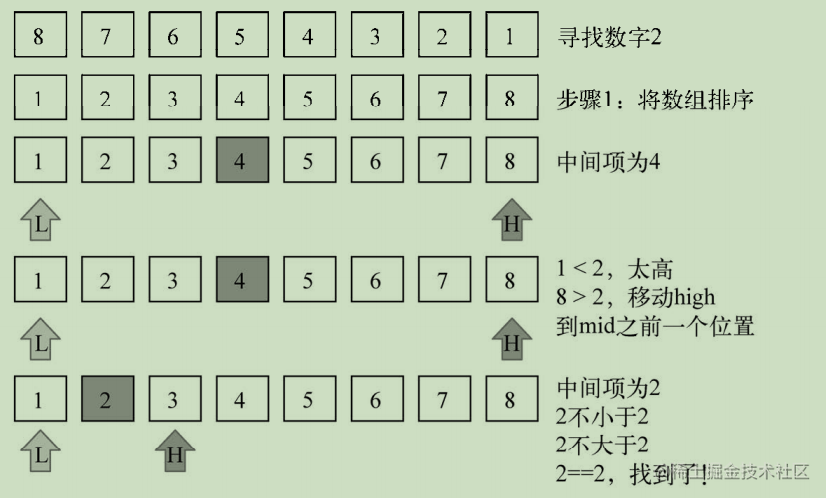### 算法模式

• 递归
• 动态规划
• 贪心算法

``````function recursiveFunction(someParam){
recursiveFunction(someParam);
};

``````function recursiveFunction1(someParam){
recursiveFunction2(someParam);
};
function recursiveFunction2(someParam){
recursiveFunction1(someParam);
};

• 它会一直执行下去(栈溢出错误)。(需要一个不再递归调用的条件)

JavaScript 调用栈大小的限制

``````var i = 0;

function recursiveFn () {
i++;
recursiveFn();
}

try {
recursiveFn();
} catch (ex) {
alert('i = ' + i + ' error: ' + ex);
// 超限错误：超过最大调用栈大小
// 内部错误：递归次数过多
}

• es6尾调用优化

### 斐波那契数列

• 1和2的斐波那契数是 1
• n（n>2）的斐波那契数是(n1)的斐波那契数加上(n2)的斐波那契数

``````// 边界条件是已知的，1和2的斐波那契数是1
function fibonacci(num){
if (num === 1 || num === 2){ //{1}
return 1;
}
}

``````function fibonacci(num){
if (num === 1 || num === 2){
return 1;
}
return fibonacci(num - 1) + fibonacci(num - 2);
}
// 当n大于2时，Fibonacci(n)等于Fibonacci(n-1)+Fibonacci(n-2)

``````function fib(num){
var n1 = 1,
n2 = 1,
n = 1;

for (var i = 3; i<=num; i++){
n = n1 + n2;
n1 = n2;
n2 = n;
}

return n;
}

• 背包问题
• 最长公共子序列
• 矩阵链相乘
• 硬币找零
• 图的全源最短路径

### 函数式编程简介

``````var printArray = function(array) {
for (var i = 0; i < array.length; i++) {
console.log(array[i]);
}
};

printArray([1, 2, 3, 4, 5]);

``````var forEach = function(array, action) {
for (var i = 0; i < array.length; i++) {
action(array[i]);
}
};

var logItem = function (item) {
console.log(item);
};

forEach([1, 2, 3, 4, 5], logItem);

1.目标是描述数据，以及要对数据应用的转换

2.程序执行顺序的重要性很低，而在命令式编程中，步骤和顺序是非常重要的

3.函数和数据集合是函数式编程的核心

4.在函数式编程中，我们可以使用和滥用函数和递归，而在命令式编程中，则使用循环、 赋值、条件和函数

• 著名的大O表示法
• 和NP完全理论

### 大 O 表示法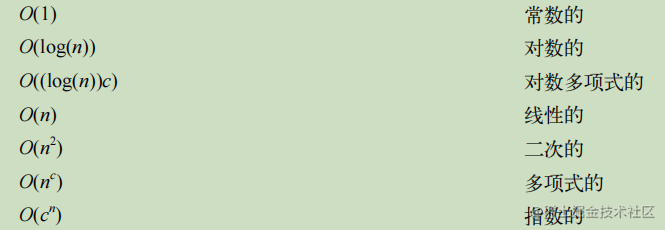• 当讨论大O表示法时，一般考虑的是CPU（时间）占用

O(1)

``````// 函数的复杂度是O(1)
// 和参数无关，increment函数的性能都一样
function increment(num){
return ++num;
}

O(n)

``````// 时间复杂度是O(n)
// n是（输入）数组的大小
function sequentialSearch(array, item){
for (var i=0; i<array.length; i++){
if (item === array[i]){ //{1}
return i;
}
}
return -1;
}

### 时间复杂度比较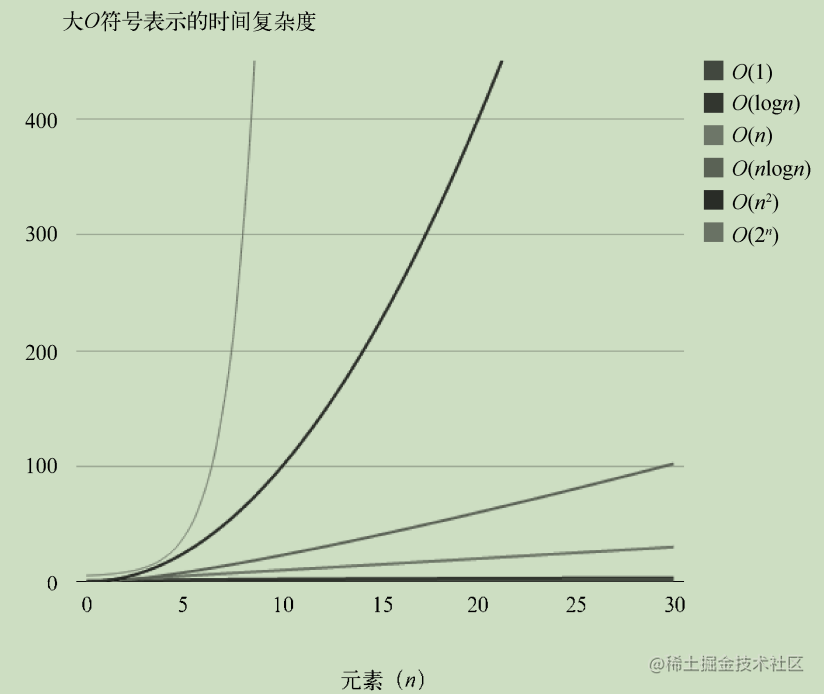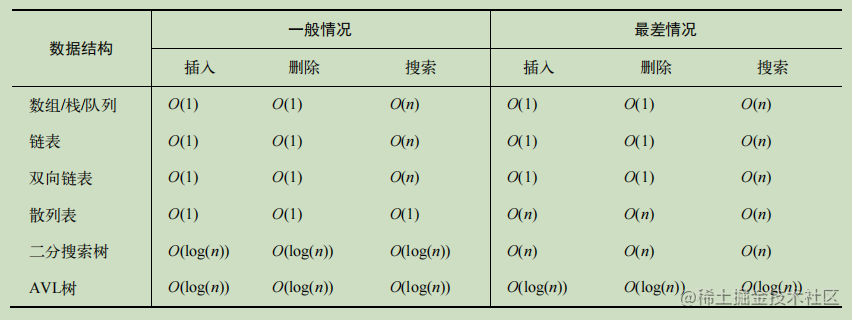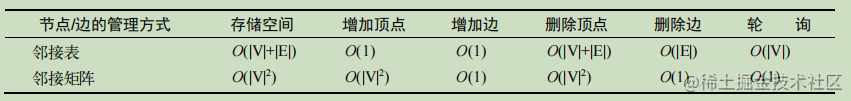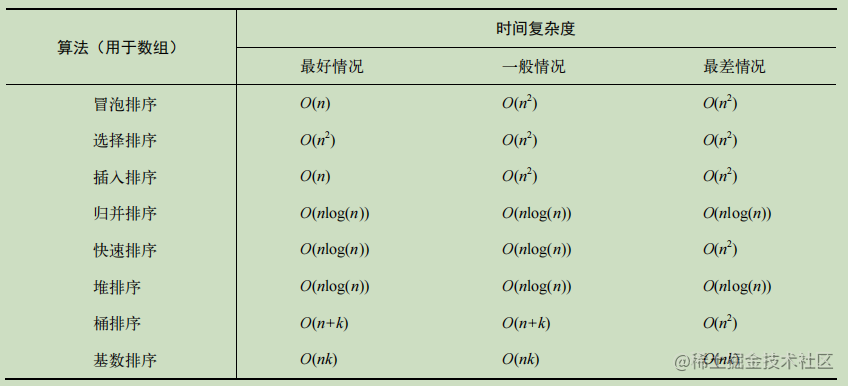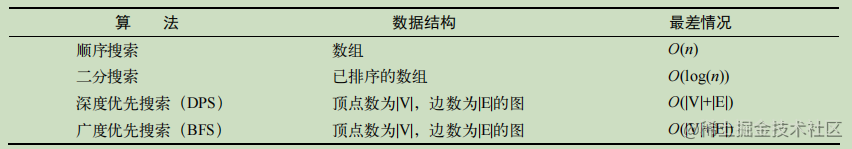### NP 完全理论

• `NP（nondeterministic polynomial，非确定性多项式）`算法
• 对于给定的问题，如果存在多项式算法，则计为`P（polynomial，多项式）`
• 如果一个问题可以在多项式时间内验证解是否正确，则计为`NP`
• `NP`问题中最难的是`NP`完全问题

1.是`NP`问题，也就是说，可以在多项式时间内验证解，但还没有找到多项式算法

2.所有的`NP`问题都能在多项式时间内归约为它

`P``NP``NP`完全和`NP`困难 问题 图：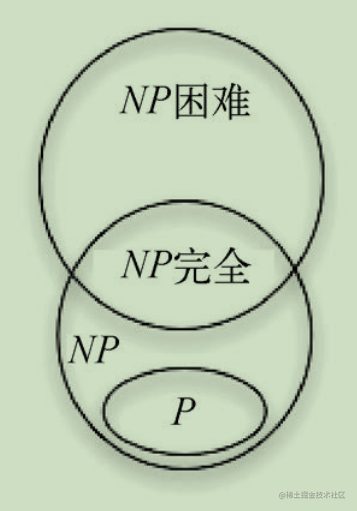❤️关注+点赞+收藏+评论+转发❤️，原创不易，鼓励笔者创作更好的文章

### 我们下期见！

`github`收录，欢迎`Star`github.com/webVueBlog/…